Theory and Modern Applications

# Existence results for fractional q-difference equations with nonlocal q-integral boundary conditions

## Abstract

In this paper, we discuss the existence of positive solutions for nonlocal q-integral boundary value problems of fractional q-difference equations. By applying the generalized Banach contraction principle, the monotone iterative method, and Krasnoselskii’s fixed point theorem, some existence results of positive solutions are obtained. In addition, some examples to illustrate our results are given.

MSC:39A13, 34B18, 34A08.

## 1 Introduction

Studies on q-difference equations appeared already at the beginning of the twentieth century in intensive works especially by Jackson , Carmichael  and other authors such as Poincare, Picard, Ramanujan. Up to date, q-difference equations have evolved into a multidisciplinary subject; for example, see  and the references therein. For some recent work on q-difference equations, we refer the reader to the papers , and basic definitions and properties of q-difference calculus can be found in the book . On the other hand, fractional differential equations have gained importance due to their numerous applications in many fields of science and engineering including fluid flow, rheology, diffusive transport akin to diffusion, electrical networks, probability, etc. For details, see [22, 23]. Many researchers studied the existence of solutions to fractional boundary value problems; see, for example,  and the references therein.

The fractional q-difference calculus had its origin in the works by Al-Salam  and Agarwal . More recently, perhaps due to the explosion in research within the fractional differential calculus setting, new developments in this theory of fractional q-difference calculus were made, specifically, q-analogues of the integral and differential fractional operators properties such as the Mittag-Leffler function, the q-Laplace transform, and q-Taylor’s formula [12, 21, 36, 37], just to mention some.

However, the theory of boundary value problems for nonlinear q-difference equations is still in the initial stage and many aspects of this theory need to be explored. Recently, there have been some paper considering the existence of solutions to boundary value problems of fractional q-difference equations, for example, [10, 1620, 38] and the references therein.

In , Ferreira considered the Dirichlet type nonlinear q-difference boundary value problem

$\left\{\begin{array}{c}{D}_{q}^{\alpha }u\left(t\right)+f\left(u\left(t\right)\right)=0,\phantom{\rule{1em}{0ex}}0

By applying a fixed point theorem in cones, sufficient conditions for the existence of nontrivial solutions were enunciated.

In , Graef and Kong investigated the boundary value problem with fractional q-derivatives

$\left\{\begin{array}{c}\left({D}_{q}^{\alpha }u\right)\left(t\right)+f\left(t,u\left(t\right)\right)=0,\phantom{\rule{1em}{0ex}}0

where $\lambda \ge 0$ is a parameter, and the uniqueness, existence, and nonexistence of positive solutions are considered in terms of different ranges of λ.

Furthermore, Ahmad, Ntouyas, and Purnaras  studied the following nonlinear fractional q-difference equation with nonlocal boundary conditions:

$\left\{\begin{array}{c}{\left(}_{C}{D}_{q}^{\alpha }u\right)\left(t\right)=f\left(t,u\left(t\right)\right),\phantom{\rule{1em}{0ex}}0\le t\le 1,1<\alpha \le 2,\hfill \\ {a}_{1}u\left(0\right)-{b}_{1}{D}_{q}u\left(0\right)={c}_{1}u\left({\eta }_{1}\right),\phantom{\rule{2em}{0ex}}{a}_{2}u\left(1\right)+{b}_{2}{D}_{q}u\left(1\right)={c}_{2}u\left({\eta }_{2}\right),\hfill \end{array}$

where ${}_{C}D_{q}^{\alpha }$ is the fractional q-derivative of the Caputo type and ${a}_{i},{b}_{i},{c}_{i},{\eta }_{i}\in \mathbf{R}$. The existence of solutions for the problem is shown by applying some well-known tools of fixed point theory such as Banach’s contraction principle, Krasnoselskii’s fixed point theorem, and the Leray-Schauder nonlinear alternative.

In this paper, we deal with the following nonlocal q-integral boundary value problem of nonlinear fractional q-derivatives equation:

$\left\{\begin{array}{c}\left({D}_{q}^{\alpha }u\right)\left(t\right)+f\left(t,u\left(t\right)\right)=0,\phantom{\rule{1em}{0ex}}t\in \left(0,1\right),\hfill \\ u\left(0\right)=0,\phantom{\rule{2em}{0ex}}u\left(1\right)=\mu {I}_{q}^{\beta }u\left(\eta \right)=\mu {\int }_{0}^{\eta }\frac{{\left(\eta -qs\right)}^{\left(\beta -1\right)}}{{\mathrm{\Gamma }}_{q}\left(\beta \right)}u\left(s\right)\phantom{\rule{0.2em}{0ex}}{d}_{q}s,\hfill \end{array}$
(1.1)

where $q\in \left(0,1\right)$, $1<\alpha \le 2$, $0<\beta \le 2$, $0<\eta <1$, and $\mu >0$ is a parameter, ${D}_{q}^{\alpha }$ is the q-derivative of Riemann-Liouville type of order α, $f:\left[0,1\right]×{\mathbf{R}}^{+}\to {\mathbf{R}}^{+}$ is continuous, in which ${\mathbf{R}}^{+}=\left[0,+\mathrm{\infty }\right)$. To the authors’ knowledge, no one has studied the existence of positive solutions for the fractional q-difference boundary value problem (1.1). In the present work, we gave the corresponding Green’s function of the boundary value problem (1.1) and its properties. By using the generalized Banach contraction principle, the monotone iterative method, and Krasnoselskii’s fixed point theorem, some existence results of positive solutions to the above boundary value problems are enunciated.

## 2 Preliminaries on q-calculus and lemmas

For the convenience of the reader, below we recall some known facts on fractional q-calculus. The presentation here can be found in, for example, [1, 21, 36, 37].

Let $q\in \left(0,1\right)$ and define

${\left[a\right]}_{q}=\frac{1-{q}^{a}}{1-q},\phantom{\rule{1em}{0ex}}a\in \mathbf{R}.$

The q-analogue of the power function ${\left(a-b\right)}^{n}$ with $n\in {\mathbf{N}}_{\mathbf{0}}:=\left\{0,1,2,\dots \right\}$ is

${\left(a-b\right)}^{\left(0\right)}=1,\phantom{\rule{2em}{0ex}}{\left(a-b\right)}^{\left(n\right)}=\prod _{k=0}^{n-1}\left(a-b{q}^{k}\right),\phantom{\rule{1em}{0ex}}n\in \mathbf{N},a,b\in \mathbf{R}.$

More generally, if $\gamma \in \mathbf{R}$, then

${\left(a-b\right)}^{\left(\gamma \right)}={a}^{\gamma }\prod _{k=0}^{\mathrm{\infty }}\frac{a-b{q}^{k}}{a-b{q}^{\gamma +k}},\phantom{\rule{1em}{0ex}}a\ne 0.$
(2.1)

Clearly, if $b=0$, then ${a}^{\left(\gamma \right)}={a}^{\gamma }$. The q-gamma function is defined by

${\mathrm{\Gamma }}_{q}\left(x\right)=\frac{{\left(1-q\right)}^{\left(x-1\right)}}{{\left(1-q\right)}^{x-1}},\phantom{\rule{1em}{0ex}}x\in \mathbf{R}\setminus \left\{0,-1,-2,\dots \right\},$

and satisfies ${\mathrm{\Gamma }}_{q}\left(x+1\right)={\left[x\right]}_{q}{\mathrm{\Gamma }}_{q}\left(x\right)$.

The q-derivative of a function f is defined by

$\left({D}_{q}f\right)\left(x\right)=\frac{f\left(qx\right)-f\left(x\right)}{\left(q-1\right)x},\phantom{\rule{2em}{0ex}}\left({D}_{q}f\right)\left(0\right)=\underset{x\to 0}{lim}\left({D}_{q}f\right)\left(x\right),$

and the q-derivatives of higher order by

$\left({D}_{q}^{0}f\right)\left(x\right)=f\left(x\right),\phantom{\rule{2em}{0ex}}\left({D}_{q}^{n}f\right)\left(x\right)={D}_{q}\left({D}_{q}^{n-1}f\right)\left(x\right),\phantom{\rule{1em}{0ex}}n\in \mathbf{N}.$

The q-integral of a function f defined in the interval $\left[0,b\right]$ is given by

$\left({I}_{q}f\right)\left(x\right)={\int }_{0}^{x}f\left(s\right)\phantom{\rule{0.2em}{0ex}}{d}_{q}s=x\left(1-q\right)\sum _{k=0}^{\mathrm{\infty }}f\left(x{q}^{k}\right){q}^{k},\phantom{\rule{1em}{0ex}}x\in \left[0,b\right],$

provided the sum converges absolutely.

If $a\in \left[0,b\right]$ and f is defined in the interval $\left[0,b\right]$, then its integral from a to b is defined by

${\int }_{a}^{b}f\left(s\right)\phantom{\rule{0.2em}{0ex}}{d}_{q}s={\int }_{0}^{b}f\left(s\right)\phantom{\rule{0.2em}{0ex}}{d}_{q}s-{\int }_{0}^{a}f\left(s\right)\phantom{\rule{0.2em}{0ex}}{d}_{q}s.$

Obviously, if $f\left(x\right)\ge g\left(x\right)$ on $\left[a,b\right]$, then ${\int }_{a}^{b}f\left(x\right)\phantom{\rule{0.2em}{0ex}}{d}_{q}x\ge {\int }_{a}^{b}g\left(x\right)\phantom{\rule{0.2em}{0ex}}{d}_{q}x$.

Similar as done for derivatives, an operator ${I}_{q}^{n}$ is given by

$\left({I}_{q}^{0}f\right)\left(x\right)=f\left(x\right),\phantom{\rule{2em}{0ex}}\left({I}_{q}^{n}f\right)\left(x\right)={I}_{q}\left({I}_{q}^{n-1}f\right)\left(x\right),\phantom{\rule{1em}{0ex}}n\in \mathbf{N}.$

The fundamental theorem of calculus applies to these operators ${I}_{q}$ and ${D}_{q}$, i.e.,

$\left({D}_{q}{I}_{q}f\right)\left(x\right)=f\left(x\right),$

and if f is continuous at $x=0$, then

$\left({I}_{q}{D}_{q}f\right)\left(x\right)=f\left(x\right)-f\left(0\right).$
(2.2)

The following formulas will be used later, namely, the integration by parts formula

${\int }_{0}^{x}f\left(s\right)\left({D}_{q}g\right)\left(s\right)\phantom{\rule{0.2em}{0ex}}{d}_{q}s={\left[f\left(s\right)g\left(s\right)\right]}_{s=0}^{s=x}-{\int }_{0}^{x}\left({D}_{q}f\right)\left(s\right)g\left(qs\right)\phantom{\rule{0.2em}{0ex}}{d}_{q}s,$

and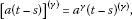(2.3)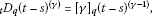(2.4)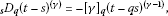(2.5)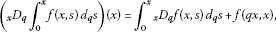(2.6)

where ${}_{t}D_{q}$ denotes the derivative with respect to the variable t.

Definition 2.1 Let $\alpha \ge 0$ and f be a function defined on $\left[0,1\right]$. The fractional q-integral of Riemann-Liouville type is $\left({I}_{q}^{0}f\right)\left(x\right)=f\left(x\right)$ and

$\left({I}_{q}^{\alpha }f\right)\left(x\right)=\frac{1}{{\mathrm{\Gamma }}_{q}\left(\alpha \right)}{\int }_{0}^{x}{\left(x-qs\right)}^{\left(\alpha -1\right)}f\left(s\right)\phantom{\rule{0.2em}{0ex}}{d}_{q}s,\phantom{\rule{1em}{0ex}}\alpha >0,x\in \left[0,1\right].$

Definition 2.2 The fractional q-derivative of the Riemann-Liouville type of order $\alpha \ge 0$ is defined by $\left({D}_{q}^{0}f\right)\left(x\right)=f\left(x\right)$ and

$\left({D}_{q}^{\alpha }f\right)\left(x\right)=\left({D}_{q}^{\left[\alpha \right]}{I}_{q}^{\left[\alpha \right]-\alpha }f\right)\left(x\right),\phantom{\rule{1em}{0ex}}\alpha >0,$

where $\left[\alpha \right]$ is the smallest integer greater than or equal to α.

Lemma 2.3 Assume that $\gamma \ge 0$ and $a\le b\le t$, then ${\left(t-a\right)}^{\left(\gamma \right)}\ge {\left(t-b\right)}^{\left(\gamma \right)}$.

Lemma 2.4 Let $\alpha ,\beta \ge 0$ and f be a function defined on $\left[0,1\right]$. Then the following formulas hold:

1. (1)

$\left({I}_{q}^{\beta }{I}_{q}^{\alpha }f\right)\left(x\right)=\left({I}_{q}^{\alpha +\beta }f\right)\left(x\right)$,

2. (2)

$\left({D}_{q}^{\alpha }{I}_{q}^{\alpha }f\right)\left(x\right)=f\left(x\right)$.

Lemma 2.5 ()

Let $\alpha >0$ and n be a positive integer. Then the following equality holds:

$\left({I}_{q}^{\alpha }{D}_{q}^{n}f\right)\left(x\right)=\left({D}_{q}^{n}{I}_{q}^{\alpha }f\right)\left(x\right)-\sum _{k=0}^{n-1}\frac{{x}^{\alpha -n+k}}{{\mathrm{\Gamma }}_{q}\left(\alpha +k-n+1\right)}\left({D}_{q}^{k}f\right)\left(0\right).$

Lemma 2.6 ()

Let $\alpha \in {\mathbf{R}}^{+}$, $\lambda \in \left(-1,+\mathrm{\infty }\right)$, the following is valid:

${I}_{q}^{\alpha }\left({\left(t-a\right)}^{\left(\lambda \right)}\right)=\frac{{\mathrm{\Gamma }}_{q}\left(\lambda +1\right)}{{\mathrm{\Gamma }}_{q}\left(\alpha +\lambda +1\right)}{\left(t-a\right)}^{\left(\alpha +\lambda \right)},\phantom{\rule{1em}{0ex}}0

Particularly, for $\lambda =0$, $a=0$, using q-integration by parts, we have

$\begin{array}{rcl}\left({I}_{q}^{\alpha }1\right)\left(t\right)& =& \frac{1}{{\mathrm{\Gamma }}_{q}\left(\alpha \right)}{\int }_{0}^{t}{\left(t-qs\right)}^{\left(\alpha -1\right)}\phantom{\rule{0.2em}{0ex}}{d}_{q}s=\frac{1}{{\mathrm{\Gamma }}_{q}\left(\alpha \right)}{\int }_{0}^{t}\frac{{}_{s}D_{q}\left({\left(t-s\right)}^{\left(\alpha \right)}\right)}{-{\left[\alpha \right]}_{q}}\phantom{\rule{0.2em}{0ex}}{d}_{q}s\\ =& -\frac{1}{{\mathrm{\Gamma }}_{q}\left(\alpha +1\right)}{{\int }_{0}^{t}}_{s}{D}_{q}\left({\left(t-s\right)}^{\left(\alpha \right)}\right)\phantom{\rule{0.2em}{0ex}}{d}_{q}s=\frac{1}{{\mathrm{\Gamma }}_{q}\left(\alpha +1\right)}{t}^{\left(\alpha \right)}.\end{array}$

Obviously, we have ${\int }_{0}^{t}{\left(t-qs\right)}^{\left(\alpha -1\right)}\phantom{\rule{0.2em}{0ex}}{d}_{q}s=\frac{1}{{\left[\alpha \right]}_{q}}{t}^{\left(\alpha \right)}$.

In order to define the solution for the problem (1.1), we need the following lemmas.

Lemma 2.7 Let $M:={\mathrm{\Gamma }}_{q}\left(\alpha +\beta \right)-\mu {\mathrm{\Gamma }}_{q}\left(\alpha \right){\eta }^{\alpha +\beta -1}>0$. Then, for a given $y\in C\left[0,1\right]$, the unique solution of the boundary value problem

$\left({D}_{q}^{\alpha }u\right)\left(t\right)+y\left(t\right)=0,\phantom{\rule{1em}{0ex}}t\in \left(0,1\right),1<\alpha \le 2,$
(2.7)

subject to the boundary condition

$\begin{array}{r}u\left(0\right)=0,\\ u\left(1\right)=\mu {I}_{q}^{\beta }u\left(\eta \right)=\mu {\int }_{0}^{\eta }\frac{{\left(\eta -qs\right)}^{\left(\beta -1\right)}}{{\mathrm{\Gamma }}_{q}\left(\beta \right)}u\left(s\right)\phantom{\rule{0.2em}{0ex}}{d}_{q}s,\phantom{\rule{1em}{0ex}}0<\beta \le 2,0<\eta <1,\end{array}$
(2.8)

is given by

$u\left(t\right)={\int }_{0}^{1}G\left(t,qs\right)y\left(s\right)\phantom{\rule{0.2em}{0ex}}{d}_{q}s,$
(2.9)

where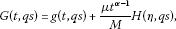(2.10)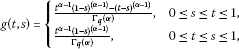(2.11)

and

$H\left(\eta ,s\right)=\left\{\begin{array}{cc}{\eta }^{\alpha +\beta -1}{\left(1-s\right)}^{\left(\alpha -1\right)}-{\left(\eta -s\right)}^{\left(\alpha +\beta -1\right)},\hfill & 0\le s\le \eta <1,\hfill \\ {\eta }^{\alpha +\beta -1}{\left(1-s\right)}^{\left(\alpha -1\right)},\hfill & 0<\eta \le s\le 1.\hfill \end{array}$
(2.12)

Proof Since $1<\alpha \le 2$, we take $n=2$. In view of Definition 2.1 and Lemma 2.4, we have

$\left({D}_{q}^{\alpha }u\right)\left(t\right)=-y\left(t\right)\phantom{\rule{1em}{0ex}}⇔\phantom{\rule{1em}{0ex}}\left({I}_{q}^{\alpha }{D}_{q}^{2}{I}_{q}^{2-\alpha }u\right)\left(t\right)=-\left({I}_{q}^{\alpha }y\right)\left(t\right).$

Then it follows from Lemma 2.5 that the solution $u\left(t\right)$ of (2.7) and (2.8) is given by

$u\left(t\right)={c}_{1}{t}^{\alpha -1}+{c}_{2}{t}^{\alpha -2}-{\int }_{0}^{t}\frac{{\left(t-qs\right)}^{\left(\alpha -1\right)}}{{\mathrm{\Gamma }}_{q}\left(\alpha \right)}y\left(s\right)\phantom{\rule{0.2em}{0ex}}{d}_{q}s$
(2.13)

for some constants ${c}_{1},{c}_{2}\in \mathbf{R}$. Since $u\left(0\right)=0$, we have ${c}_{2}=0$.

Using the Riemann-Liouville integral of order β for (2.13), we have

$\begin{array}{rcl}\left({I}_{q}^{\beta }u\right)\left(t\right)& =& {\int }_{0}^{t}\frac{{\left(t-qs\right)}^{\left(\beta -1\right)}}{{\mathrm{\Gamma }}_{q}\left(\beta \right)}\left({c}_{1}{s}^{\alpha -1}-{\int }_{0}^{s}\frac{{\left(s-qx\right)}^{\left(\alpha -1\right)}}{{\mathrm{\Gamma }}_{q}\left(\alpha \right)}y\left(x\right)\phantom{\rule{0.2em}{0ex}}{d}_{q}x\right)\phantom{\rule{0.2em}{0ex}}{d}_{q}s\\ =& -{\int }_{0}^{t}\frac{{\left(t-qs\right)}^{\left(\alpha +\beta -1\right)}}{{\mathrm{\Gamma }}_{q}\left(\alpha +\beta \right)}y\left(s\right)\phantom{\rule{0.2em}{0ex}}{d}_{q}s+{c}_{1}{\int }_{0}^{t}\frac{{\left(t-qs\right)}^{\left(\beta -1\right)}}{{\mathrm{\Gamma }}_{q}\left(\beta \right)}{s}^{\alpha -1}\phantom{\rule{0.2em}{0ex}}{d}_{q}s\\ =& -{\int }_{0}^{t}\frac{{\left(t-qs\right)}^{\left(\alpha +\beta -1\right)}}{{\mathrm{\Gamma }}_{q}\left(\alpha +\beta \right)}y\left(s\right)\phantom{\rule{0.2em}{0ex}}{d}_{q}s+{c}_{1}\frac{{\mathrm{\Gamma }}_{q}\left(\alpha \right)}{{\mathrm{\Gamma }}_{q}\left(\alpha +\beta \right)}{t}^{\alpha +\beta -1},\end{array}$

where we have used Lemma 2.4 and Lemma 2.6. Using the boundary condition $u\left(1\right)=\mu {I}_{q}^{\beta }u\left(\eta \right)$, we get

${c}_{1}=\frac{{\mathrm{\Gamma }}_{q}\left(\alpha +\beta \right)}{M}\left({\int }_{0}^{1}\frac{{\left(1-qs\right)}^{\left(\alpha -1\right)}}{{\mathrm{\Gamma }}_{q}\left(\alpha \right)}y\left(s\right)\phantom{\rule{0.2em}{0ex}}{d}_{q}s-\mu {\int }_{0}^{\eta }\frac{{\left(\eta -qs\right)}^{\left(\alpha +\beta -1\right)}}{{\mathrm{\Gamma }}_{q}\left(\alpha +\beta \right)}y\left(s\right)\phantom{\rule{0.2em}{0ex}}{d}_{q}s\right).$

Hence, we have

$\begin{array}{rcl}u\left(t\right)& =& \frac{{\mathrm{\Gamma }}_{q}\left(\alpha +\beta \right){t}^{\alpha -1}}{M}\left({\int }_{0}^{1}\frac{{\left(1-qs\right)}^{\left(\alpha -1\right)}}{{\mathrm{\Gamma }}_{q}\left(\alpha \right)}y\left(s\right)\phantom{\rule{0.2em}{0ex}}{d}_{q}s-\mu {\int }_{0}^{\eta }\frac{{\left(\eta -qs\right)}^{\left(\alpha +\beta -1\right)}}{{\mathrm{\Gamma }}_{q}\left(\alpha +\beta \right)}y\left(s\right)\phantom{\rule{0.2em}{0ex}}{d}_{q}s\right)\\ -{\int }_{0}^{t}\frac{{\left(t-qs\right)}^{\left(\alpha -1\right)}}{{\mathrm{\Gamma }}_{q}\left(\alpha \right)}y\left(s\right)\phantom{\rule{0.2em}{0ex}}{d}_{q}s\\ =& {\int }_{0}^{1}\frac{{t}^{\alpha -1}{\left(1-qs\right)}^{\left(\alpha -1\right)}}{{\mathrm{\Gamma }}_{q}\left(\alpha \right)}y\left(s\right)\phantom{\rule{0.2em}{0ex}}{d}_{q}s-{\int }_{0}^{t}\frac{{\left(t-qs\right)}^{\left(\alpha -1\right)}}{{\mathrm{\Gamma }}_{q}\left(\alpha \right)}y\left(s\right)\phantom{\rule{0.2em}{0ex}}{d}_{q}s\\ +\frac{\mu {t}^{\alpha -1}}{M}\left({\int }_{0}^{1}{\eta }^{\alpha +\beta -1}{\left(1-qs\right)}^{\left(\alpha -1\right)}y\left(s\right)\phantom{\rule{0.2em}{0ex}}{d}_{q}s-{\int }_{0}^{\eta }{\left(\eta -qs\right)}^{\left(\alpha +\beta -1\right)}y\left(s\right)\phantom{\rule{0.2em}{0ex}}{d}_{q}s\right)\\ =& {\int }_{0}^{1}g\left(t,qs\right)y\left(s\right)\phantom{\rule{0.2em}{0ex}}{d}_{q}s+\frac{\mu {t}^{\alpha -1}}{M}{\int }_{0}^{1}H\left(\eta ,qs\right)\phantom{\rule{0.2em}{0ex}}{d}_{q}s\\ =& {\int }_{0}^{1}G\left(t,qs\right)y\left(s\right)\phantom{\rule{0.2em}{0ex}}{d}_{q}s.\end{array}$

This completes the proof of the lemma. □

Remark 2.8 For the special case where $\mu =0$, Lemma 2.7 has been obtained by Ferreira .

Lemma 2.9 ()

The function $g\left(t,s\right)$ defined by (2.11) satisfies the following properties:

$g\left(t,qs\right)\ge 0\phantom{\rule{1em}{0ex}}\mathit{\text{and}}\phantom{\rule{1em}{0ex}}g\left(t,qs\right)\le g\left(qs,qs\right)\phantom{\rule{1em}{0ex}}\mathit{\text{for all}}0\le t,s\le 1.$

Lemma 2.10 The function $G\left(t,qs\right)$ defined by (2.10) satisfies the following properties:

1. (i)

G is a continuous function and $G\left(t,qs\right)\ge 0$ for $\left(t,s\right)\in \left[0,1\right]×\left[0,1\right]$.

2. (ii)

There exists a positive function $\rho \in C\left(\left(0,1\right),\left(0,+\mathrm{\infty }\right)\right)$ such that

$\underset{0\le t\le 1}{max}G\left(t,qs\right)\le \rho \left(s\right),$

where

$\rho \left(s\right)=g\left(qs,qs\right)+\frac{\mu }{M}H\left(\eta ,qs\right),\phantom{\rule{1em}{0ex}}s\in \left(0,1\right).$

Proof It is easy to prove that the statement (i) holds. On the other hand, we note that $g\left(t,s\right)$ defined by (2.11) is decreasing with respect to t for $s\le t$ and increasing with respect to t for $t\le s$. Hence, we have

$\begin{array}{rcl}\underset{0\le t\le 1}{max}G\left(t,qs\right)& =& \underset{0\le t\le 1}{max}\left(g\left(t,qs\right)+\frac{\mu {t}^{\alpha -1}}{M}H\left(\eta ,qs\right)\right)\\ \le & g\left(qs,qs\right)+\frac{\mu }{M}H\left(\eta ,qs\right):=\rho \left(s\right),\phantom{\rule{1em}{0ex}}s\in \left(0,1\right).\end{array}$

The proof is completed. □

## 3 The main results

Let $X=C\left(\left[0,1\right]\right)$ be a Banach space endowed with the norm ${\parallel u\parallel }_{X}={max}_{0\le t\le 1}|u\left(t\right)|$. Define the cone $P\subset X$ by $P=\left\{u\in X:u\left(t\right)\ge 0,0\le t\le 1\right\}$.

Define the operator $T:P\to X$ as follows:

$\left(Tu\right)\left(t\right)={\int }_{0}^{1}G\left(t,qs\right)f\left(s,u\left(s\right)\right)\phantom{\rule{0.2em}{0ex}}{d}_{q}s.$
(3.1)

It follows from the nonnegativeness and continuity of G and f that the operator $T:P\to X$ satisfies $T\left(P\right)\subset P$ and is completely continuous.

Theorem 3.1 Suppose that $f:\left[0,1\right]×{\mathbf{R}}^{+}\to {\mathbf{R}}^{+}$ is continuous and there exists a function $h:\left[0,1\right]\to {\mathbf{R}}^{+}$ such that

$|f\left(t,u\right)-f\left(t,v\right)|\le h\left(t\right)|u-v|,\phantom{\rule{1em}{0ex}}t\in \left[0,1\right],u,v\in {\mathbf{R}}^{+}.$
(3.2)

Then the BVP (1.1) has a unique positive solution provided

${\int }_{0}^{1}{s}^{\alpha -1}{\left(1-qs\right)}^{\left(\alpha -1\right)}h\left(s\right)\phantom{\rule{0.2em}{0ex}}{d}_{q}s<\frac{{\mathrm{\Gamma }}_{q}\left(\alpha \right)M}{2{\mathrm{\Gamma }}_{q}\left(\alpha +\beta \right)}.$
(3.3)

Proof We will prove that under the assumptions (3.2) and (3.3), ${T}^{m}$ is a contraction operator for m sufficiently large.

By (2.10), (2.11), and (2.12), for $u,v\in P$, we obtain the estimate

$\begin{array}{rcl}|\left(Tu\right)\left(t\right)-\left(Tv\right)\left(t\right)|& \le & {\int }_{0}^{1}G\left(t,qs\right)|f\left(s,u\left(s\right)\right)-f\left(s,v\left(s\right)\right)|\phantom{\rule{0.2em}{0ex}}{d}_{q}s\\ =& {\int }_{0}^{1}\left[g\left(t,qs\right)+\frac{\mu {t}^{\alpha -1}}{M}H\left(\eta ,qs\right)\right]|f\left(s,u\left(s\right)\right)-f\left(s,v\left(s\right)\right)|\phantom{\rule{0.2em}{0ex}}{d}_{q}s\\ \le & {\int }_{0}^{1}\frac{{t}^{\alpha -1}{\left(1-qs\right)}^{\left(\alpha -1\right)}}{{\mathrm{\Gamma }}_{q}\left(\alpha \right)}h\left(s\right)\phantom{\rule{0.2em}{0ex}}{d}_{q}s\cdot {\parallel u-v\parallel }_{X}\\ +\mu {\eta }^{\alpha +\beta -1}{\int }_{0}^{1}\frac{{t}^{\alpha -1}{\left(1-qs\right)}^{\left(\alpha -1\right)}}{M}h\left(s\right)\phantom{\rule{0.2em}{0ex}}{d}_{q}s\cdot {\parallel u-v\parallel }_{X}\\ =& \frac{{\mathrm{\Gamma }}_{q}\left(\alpha +\beta \right){t}^{\alpha -1}{\parallel u-v\parallel }_{X}}{{\mathrm{\Gamma }}_{q}\left(\alpha \right)M}{\int }_{0}^{1}{\left(1-qs\right)}^{\left(\alpha -1\right)}h\left(s\right)\phantom{\rule{0.2em}{0ex}}{d}_{q}s\\ =& \frac{{\mathrm{\Lambda }}_{1}{\mathrm{\Gamma }}_{q}\left(\alpha +\beta \right){t}^{\alpha -1}}{{\mathrm{\Gamma }}_{q}\left(\alpha \right)M}{\parallel u-v\parallel }_{X},\end{array}$

where ${\mathrm{\Lambda }}_{1}={\int }_{0}^{1}{\left(1-qs\right)}^{\left(\alpha -1\right)}h\left(s\right)\phantom{\rule{0.2em}{0ex}}{d}_{q}s$.

Consequently,

$\begin{array}{rcl}|\left({T}^{2}u\right)\left(t\right)-\left({T}^{2}v\right)\left(t\right)|& \le & {\int }_{0}^{1}G\left(t,qs\right)|f\left(s,\left(Tu\right)\left(s\right)\right)-f\left(s,\left(Tv\right)\left(s\right)\right)|\phantom{\rule{0.2em}{0ex}}{d}_{q}s\\ \le & \frac{{\mathrm{\Lambda }}_{1}{\mathrm{\Gamma }}_{q}\left(\alpha +\beta \right){\parallel u-v\parallel }_{X}}{{\mathrm{\Gamma }}_{q}\left(\alpha \right)M}{\int }_{0}^{1}G\left(t,qs\right){s}^{\alpha -1}h\left(s\right)\phantom{\rule{0.2em}{0ex}}{d}_{q}s\\ \le & \frac{{\mathrm{\Lambda }}_{1}{\left[{\mathrm{\Gamma }}_{q}\left(\alpha +\beta \right)\right]}^{2}{t}^{\alpha -1}{\parallel u-v\parallel }_{X}}{{\left[{\mathrm{\Gamma }}_{q}\left(\alpha \right)M\right]}^{2}}{\int }_{0}^{1}{s}^{\alpha -1}{\left(1-qs\right)}^{\left(\alpha -1\right)}h\left(s\right)\phantom{\rule{0.2em}{0ex}}{d}_{q}s\\ =& \frac{{\mathrm{\Lambda }}_{1}{\mathrm{\Lambda }}_{2}{\left[{\mathrm{\Gamma }}_{q}\left(\alpha +\beta \right)\right]}^{2}{t}^{\alpha -1}}{{\left[{\mathrm{\Gamma }}_{q}\left(\alpha \right)M\right]}^{2}}{\parallel u-v\parallel }_{X},\end{array}$

where ${\mathrm{\Lambda }}_{2}={\int }_{0}^{1}{s}^{\alpha -1}{\left(1-qs\right)}^{\left(\alpha -1\right)}h\left(s\right)\phantom{\rule{0.2em}{0ex}}{d}_{q}s$.

By introduction, we have

$|\left({T}^{m}u\right)\left(t\right)-\left({T}^{m}v\right)\left(t\right)|\le \frac{{\mathrm{\Lambda }}_{1}{\mathrm{\Lambda }}_{2}^{m-1}{\left[{\mathrm{\Gamma }}_{q}\left(\alpha +\beta \right)\right]}^{m}{t}^{\alpha -1}}{{\left[{\mathrm{\Gamma }}_{q}\left(\alpha \right)M\right]}^{m}}{\parallel u-v\parallel }_{X}.$

According to (3.3), we can choose m sufficiently large such that

$\frac{{\mathrm{\Lambda }}_{1}{\mathrm{\Lambda }}_{2}^{m-1}{\left[{\mathrm{\Gamma }}_{q}\left(\alpha +\beta \right)\right]}^{m}}{{\left[{\mathrm{\Gamma }}_{q}\left(\alpha \right)M\right]}^{m}}=\frac{{\mathrm{\Lambda }}_{1}}{{\mathrm{\Lambda }}_{2}}{\left[\frac{{\mathrm{\Lambda }}_{2}{\mathrm{\Gamma }}_{q}\left(\alpha +\beta \right)}{{\mathrm{\Gamma }}_{q}\left(\alpha \right)M}\right]}^{m}<{\left(\frac{1}{2}\right)}^{m}\frac{{\mathrm{\Lambda }}_{1}}{{\mathrm{\Lambda }}_{2}}<\frac{1}{2},$

which implies

${\parallel \left({T}^{m}u\right)\left(t\right)-\left({T}^{m}v\right)\left(t\right)\parallel }_{X}<\frac{1}{2}{\parallel u-v\parallel }_{X}.$

Hence, it follows from the generalized Banach contraction principle that the BVP (1.1) has a unique positive solution. □

Remark 3.2 When $h\left(t\right)\equiv {h}_{0}$ is a constant, the condition (3.2) reduces to a Lipschitz condition.

For the sake of convenience, we set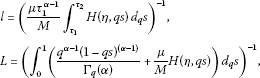where ${\tau }_{1}={q}^{{m}_{1}}$, ${\tau }_{2}={q}^{{m}_{2}}$ with ${m}_{1},{m}_{2}\in {\mathbf{N}}_{\mathbf{0}}$, ${m}_{1}>{m}_{2}$.

Theorem 3.3 Suppose that there exists $0<{\xi }_{1}<{\xi }_{2}$ such that

(${H}_{f}$) $f\left(t,u\right):\left[0,1\right]×\left[0,{\xi }_{2}\right]\to {\mathbf{R}}^{+}$ is continuous and nondecreasing relative to u, and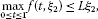(3.4)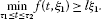(3.5)

Then the BVP (1.1) has one positive solution ${u}^{\ast }$ satisfying

${\xi }_{1}\le {\parallel {u}^{\ast }\parallel }_{X}\le {\xi }_{2}\phantom{\rule{1em}{0ex}}\mathit{\text{and}}\phantom{\rule{1em}{0ex}}\underset{m\to \mathrm{\infty }}{lim}{T}^{m}{u}_{0}={u}^{\ast },\phantom{\rule{2em}{0ex}}{u}_{0}\left(t\right)={\xi }_{2}.$

Proof We denote $\mathrm{\Omega }\left[{\xi }_{1},{\xi }_{2}\right]=\left\{u\in P:{\xi }_{1}\le u\le {\xi }_{2}\right\}$. In what follows, we first show that $T:\mathrm{\Omega }\left[{\xi }_{1},{\xi }_{2}\right]\to \mathrm{\Omega }\left[{\xi }_{1},{\xi }_{2}\right]$.

Let $u\in \mathrm{\Omega }\left[{\xi }_{1},{\xi }_{2}\right]$; then $0<{\xi }_{1}\le u\left(t\right)\le {\parallel u\parallel }_{X}\le {\xi }_{2}$. By assumption (${H}_{f}$), we haveHence, for any $u\in \mathrm{\Omega }\left[{\xi }_{1},{\xi }_{2}\right]$,

$\begin{array}{rcl}{\parallel Tu\parallel }_{X}& =& \underset{0\le t\le 1}{max}{\int }_{0}^{1}|G\left(t,qs\right)f\left(s,u\left(s\right)\right)|\phantom{\rule{0.2em}{0ex}}{d}_{q}s\\ \le & {\int }_{0}^{1}\rho \left(s\right)f\left(s,u\left(s\right)\right)\phantom{\rule{0.2em}{0ex}}{d}_{q}s\\ \le & L{\xi }_{2}{\int }_{0}^{1}\rho \left(s\right)\phantom{\rule{0.2em}{0ex}}{d}_{q}s\\ \le & L{\xi }_{2}{\int }_{0}^{1}\left(\frac{{q}^{\alpha -1}{\left(1-qs\right)}^{\left(\alpha -1\right)}}{{\mathrm{\Gamma }}_{q}\left(\alpha \right)}+\frac{\mu }{M}H\left(\eta ,qs\right)\right)\phantom{\rule{0.2em}{0ex}}{d}_{q}s={\xi }_{2},\end{array}$

and

$\begin{array}{rcl}{\parallel Tu\parallel }_{X}& \ge & \underset{{\tau }_{1}\le t\le {\tau }_{2}}{min}\left({\int }_{0}^{1}|g\left(t,qs\right)f\left(s,u\left(s\right)\right)|\phantom{\rule{0.2em}{0ex}}{d}_{q}s+\frac{\mu {t}^{\alpha -1}}{M}{\int }_{0}^{1}|H\left(\eta ,qs\right)f\left(s,u\left(s\right)\right)|\phantom{\rule{0.2em}{0ex}}{d}_{q}s\right)\\ \ge & \frac{\mu {\tau }_{1}^{\alpha -1}}{M}\left({\int }_{{\tau }_{1}}^{{\tau }_{2}}H\left(\eta ,qs\right)\phantom{\rule{0.2em}{0ex}}{d}_{q}s\right)\cdot l{\xi }_{1}={\xi }_{1}.\end{array}$

Thus, we get $T:\mathrm{\Omega }\left[{\xi }_{1},{\xi }_{2}\right]\to \mathrm{\Omega }\left[{\xi }_{1},{\xi }_{2}\right]$.

Let ${u}_{0}\left(t\right)={\xi }_{2}$, $0\le t\le 1$; then ${u}_{0}\in \mathrm{\Omega }\left[{\xi }_{1},{\xi }_{2}\right]$. Let ${u}_{1}\left(t\right)=T{u}_{0}\left(t\right)$; then ${u}_{1}\in \mathrm{\Omega }\left[{\xi }_{1},{\xi }_{2}\right]$. We denote ${u}_{m+1}=T{u}_{m}={T}^{m+1}{u}_{0}$, $m=0,1,2,\dots$ . According to $T:\mathrm{\Omega }\left[{\xi }_{1},{\xi }_{2}\right]\to \mathrm{\Omega }\left[{\xi }_{1},{\xi }_{2}\right]$, we have ${u}_{n}\in \mathrm{\Omega }\left[{\xi }_{1},{\xi }_{2}\right]$, $n=0,1,2,\dots$ . Since T is completely continuous, we assert that ${\left\{{u}_{m}\right\}}_{m=1}^{\mathrm{\infty }}$ has a convergent subsequence ${\left\{{u}_{{m}_{k}}\right\}}_{k=1}^{\mathrm{\infty }}$ and there exists ${u}^{\ast }\in \mathrm{\Omega }\left[{\xi }_{1},{\xi }_{2}\right]$ such that ${lim}_{k\to +\mathrm{\infty }}{u}_{{m}_{k}}={u}^{\ast }$. Since ${u}_{1}\in \mathrm{\Omega }\left[{\xi }_{1},{\xi }_{2}\right]$, then ${\xi }_{1}\le {u}_{1}\le {\parallel {u}_{1}\parallel }_{X}\le {\xi }_{2}={u}_{0}$. According to the definition of T and (${H}_{f}$), we have

$\begin{array}{rcl}{u}_{1}\left(t\right)& =& \left(T{u}_{0}\right)\left(t\right)\\ =& {\int }_{0}^{1}G\left(t,qs\right)f\left(s,{u}_{0}\left(s\right)\right)\phantom{\rule{0.2em}{0ex}}{d}_{q}s\\ \le & {\int }_{0}^{1}\rho \left(s\right)f\left(s,{\xi }_{2}\right)\phantom{\rule{0.2em}{0ex}}{d}_{q}s\\ \le & L{\xi }_{2}{\int }_{0}^{1}\left(\frac{{q}^{\alpha -1}{\left(1-qs\right)}^{\left(\alpha -1\right)}}{{\mathrm{\Gamma }}_{q}\left(\alpha \right)}+\frac{\mu }{M}H\left(\eta ,qs\right)\right)\phantom{\rule{0.2em}{0ex}}{d}_{q}s={\xi }_{2}={u}_{0}\left(t\right),\end{array}$

which implies

${u}_{2}\left(t\right)=T{u}_{1}\left(t\right)\le T{u}_{0}\left(t\right)={u}_{1}\left(t\right),\phantom{\rule{1em}{0ex}}0\le t\le 1.$

By introduction, we have ${u}_{m+1}\left(t\right)\le {u}_{m}\left(t\right)$ for $0\le t\le 1$, $m=0,1,2,\dots$ . Thus, there exists ${u}^{\ast }\in \mathrm{\Omega }\left[{\xi }_{1},{\xi }_{2}\right]$ such that ${lim}_{m\to +\mathrm{\infty }}{u}_{m}={u}^{\ast }$. From the continuity of T and ${u}_{m+1}=T{u}_{m}$, we have ${u}^{\ast }=T{u}^{\ast }$. The proof is completed. □

Our next existence result is based on Krasnoselskii’s fixed point theorem .

Lemma 3.4 (Krasnoselskii’s)

Let E be a Banach space, and let $P\subset E$ be a cone. Assume ${\mathrm{\Omega }}_{1}$, ${\mathrm{\Omega }}_{2}$ are open subsets of E with $\theta \in {\mathrm{\Omega }}_{1}\subset {\overline{\mathrm{\Omega }}}_{1}\subset {\mathrm{\Omega }}_{2}$, and let $T:P\cap \left({\overline{\mathrm{\Omega }}}_{2}\setminus {\mathrm{\Omega }}_{1}\right)\to P$ be a completely continuous operator such that

$\parallel Tu\parallel \ge \parallel u\parallel ,\phantom{\rule{1em}{0ex}}u\in P\cap \partial {\mathrm{\Omega }}_{1}\phantom{\rule{1em}{0ex}}\mathit{\text{and}}\phantom{\rule{1em}{0ex}}\parallel Tu\parallel \le \parallel u\parallel ,\phantom{\rule{1em}{0ex}}u\in P\cap \partial {\mathrm{\Omega }}_{2}.$

Then T has at least one fixed point in $P\cap \left({\overline{\mathrm{\Omega }}}_{2}\setminus {\mathrm{\Omega }}_{1}\right)$.

Theorem 3.5 Let $f\left(t,u\right)$ be a nonnegative continuous function on $\left[0,1\right]×{\mathbf{R}}^{+}$. In addition, we assume that

(H1) There exists a positive constant ${r}_{1}$ such that

$f\left(t,u\right)\ge \kappa {r}_{1},\phantom{\rule{1em}{0ex}}\mathit{\text{for}}\left(t,u\right)\in \left[{\tau }_{3},{\tau }_{4}\right]×\left[0,{r}_{1}\right],$

where ${\tau }_{3}={q}^{{m}_{3}}$, ${\tau }_{4}={q}^{{m}_{4}}$ with ${m}_{3},{m}_{4}\in {\mathbf{N}}_{\mathbf{0}}$, ${m}_{3}>{m}_{4}$, and

$\kappa \ge {\left({\int }_{{\tau }_{3}}^{{\tau }_{4}}\left(g\left(qs,qs\right)+\frac{\mu }{M}H\left(\eta ,qs\right)\right)\phantom{\rule{0.2em}{0ex}}{d}_{q}s\right)}^{-1}.$

(H2) There exists a positive constant ${r}_{2}$ with ${r}_{2}>{r}_{1}$ such that

$f\left(t,u\right)\le L{r}_{2},\phantom{\rule{1em}{0ex}}\mathit{\text{for}}\left(t,u\right)\in \left[0,1\right]×\left[0,{r}_{2}\right].$

Then the BVP (1.1) has at least one positive solution ${u}_{0}$ satisfying $0<{r}_{1}\le {\parallel {u}_{0}\parallel }_{X}\le {r}_{2}$.

Proof By Lemma 2.9, we obtain that ${max}_{0\le t\le 1}g\left(t,qs\right)=g\left(qs,qs\right)$. Let ${\mathrm{\Omega }}_{1}=\left\{u\in X:{\parallel u\parallel }_{X}<{r}_{1}\right\}$. For any $u\in X\cap \partial {\mathrm{\Omega }}_{1}$, according to (H1) and the definitions of ${\tau }_{3}$ and ${\tau }_{4}$, we obtain

$\begin{array}{rcl}{\parallel Tu\parallel }_{X}& =& \underset{0\le t\le 1}{max}{\int }_{0}^{1}g\left(t,qs\right)f\left(s,u\left(s\right)\right)\phantom{\rule{0.2em}{0ex}}{d}_{q}s+\underset{0\le t\le 1}{max}{\int }_{0}^{1}\frac{\mu {t}^{\alpha -1}}{M}H\left(\eta ,qs\right)f\left(s,u\left(s\right)\right)\phantom{\rule{0.2em}{0ex}}{d}_{q}s\\ =& {\int }_{0}^{1}\left[g\left(qs,qs\right)+\frac{\mu }{M}H\left(\eta ,qs\right)\right]f\left(s,u\left(s\right)\right)\phantom{\rule{0.2em}{0ex}}{d}_{q}s\\ \ge & {\int }_{{\tau }_{3}}^{{\tau }_{4}}\left(g\left(qs,qs\right)+\frac{\mu }{M}H\left(\eta ,qs\right)\right)f\left(s,u\left(s\right)\right)\phantom{\rule{0.2em}{0ex}}{d}_{q}s\\ \ge & \kappa {r}_{1}{\int }_{{\tau }_{3}}^{{\tau }_{4}}\left(g\left(qs,qs\right)+\frac{\mu }{M}H\left(\eta ,qs\right)\right)\phantom{\rule{0.2em}{0ex}}{d}_{q}s\\ \ge & {\parallel u\parallel }_{X}={r}_{1}.\end{array}$

Let ${\mathrm{\Omega }}_{2}=\left\{u\in X:{\parallel u\parallel }_{X}<{r}_{2}\right\}$. For any $u\in X\cap \partial {\mathrm{\Omega }}_{2}$, by (H2) and Lemma 2.10, we have

$\begin{array}{rcl}{\parallel Tu\parallel }_{X}& =& \underset{0\le t\le 1}{max}{\int }_{0}^{1}G\left(t,qs\right)f\left(s,u\left(s\right)\right)\phantom{\rule{0.2em}{0ex}}{d}_{q}s\\ \le & L{r}_{2}{\int }_{0}^{1}\rho \left(s\right)\phantom{\rule{0.2em}{0ex}}{d}_{q}s\\ \le & L{r}_{2}{\int }_{0}^{1}\left(\frac{{q}^{\alpha -1}{\left(1-qs\right)}^{\left(\alpha -1\right)}}{{\mathrm{\Gamma }}_{q}\left(\alpha \right)}+\frac{\mu }{M}H\left(\eta ,qs\right)\right)\phantom{\rule{0.2em}{0ex}}{d}_{q}s={\parallel u\parallel }_{X}={r}_{2}.\end{array}$

Now, an application of Lemma 3.4 concludes the proof. □

Theorem 3.6 Assume that there exist $m+1$ positive numbers $0<{r}_{1}<{r}_{2}<\cdots <{r}_{m+1}$ such that

(H3) $f\left(t,u\right)>\kappa {r}_{2j-1}$ for $\left(t,u\right)\in \left[{\tau }_{3},{\tau }_{4}\right]×\left[0,{r}_{2j-1}\right]$ and $f\left(t,u\right) for $\left(t,u\right)\in \left[0,1\right]×\left[0,{r}_{2j}\right]$, $j=1,2,\dots ,\left[\frac{m+1}{2}\right]$; or

(H4) $f\left(t,u\right)>\kappa {r}_{2j}$ for $\left(t,u\right)\in \left[{\tau }_{3},{\tau }_{4}\right]×\left[0,{r}_{2j}\right]$ and $f\left(t,u\right) for $\left(t,u\right)\in \left[0,1\right]×\left[0,{r}_{2j-1}\right]$, $j=1,2,\dots ,\left[\frac{m+1}{2}\right]$,

where ${\tau }_{3}$, ${\tau }_{4}$, κ are given in (H1).

Then the BVP (1.1) has at least n positive solutions ${u}_{1},{u}_{2},\dots ,{u}_{m}$ with ${r}_{j}<{\parallel {u}_{j}\parallel }_{X}<{r}_{j+1}$, $j=1,\dots ,m$.

Proof Suppose that the condition (H3) holds. According to the continuity of f, for every pair $\left({r}_{j},{r}_{j+1}\right)$, there exists $\left({a}_{j},{b}_{j}\right)$ with ${r}_{j}<{a}_{j}<{b}_{j}<{r}_{j+1}$ such that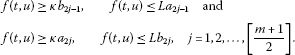It follows from Theorem 3.5 that every pair $\left({a}_{j},{b}_{j}\right)$ presents a positive solution of the BVP (1.1) such that ${a}_{j}\le {\parallel {u}_{j}\parallel }_{X}<{b}_{j}$, $j=1,2,\dots ,m$.

When the condition (H4) holds, the proofs are similar to those in the case (H3). The proof is completed. □

## 4 Examples

Example 4.1

The fractional boundary value problem

$\left\{\begin{array}{c}{D}_{0.5}^{1.5}u\left(t\right)+\frac{{e}^{t}\left(1+u\right)}{2\left(1+{e}^{t}\right)}+t{sin}^{2}t+4=0,\phantom{\rule{1em}{0ex}}0
(4.1)

has a unique positive solution.

Proof In this case, $\alpha =1.5$, $\beta =q=0.5$, $\mu =0.25$, $\eta =0.75$. Let

$f\left(t,u\right)=\frac{{e}^{t}\left(1+u\right)}{2\left(1+{e}^{t}\right)}+t{sin}^{2}t+4,\phantom{\rule{1em}{0ex}}\left(t,u\right)\in \left[0,1\right]×\left[0,+\mathrm{\infty }\right),$

and $h\left(t\right)=\frac{{e}^{t}}{2\left(1+{e}^{t}\right)}$. It is easy to prove that

By simple calculation, we get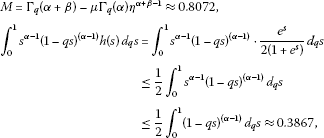and

$\begin{array}{rcl}{\int }_{0}^{1}{\left(1-qs\right)}^{\left(\alpha -1\right)}h\left(s\right)\phantom{\rule{0.2em}{0ex}}{d}_{q}s& =& {\int }_{0}^{1}{\left(1-qs\right)}^{\left(\alpha -1\right)}\cdot \frac{{e}^{s}}{2\left(1+{e}^{s}\right)}\phantom{\rule{0.2em}{0ex}}{d}_{q}s\\ \le & \frac{1}{2}{\int }_{0}^{1}{\left(1-qs\right)}^{\left(\alpha -1\right)}\phantom{\rule{0.2em}{0ex}}{d}_{q}s\approx 0.3867,\end{array}$

which implies that

${\int }_{0}^{1}{s}^{\alpha -1}{\left(1-qs\right)}^{\left(\alpha -1\right)}h\left(s\right)\phantom{\rule{0.2em}{0ex}}{d}_{q}s<\frac{{\mathrm{\Gamma }}_{q}\left(\alpha \right)M}{2{\mathrm{\Gamma }}_{q}\left(\alpha +\beta \right)}\approx 0.4512.$

Obviously, for any $m\ge 3$, we have

$\frac{{\mathrm{\Lambda }}_{1}{\mathrm{\Lambda }}_{2}^{m-1}{\left[{\mathrm{\Gamma }}_{q}\left(\alpha +\beta \right)\right]}^{m}}{{\left[{\mathrm{\Gamma }}_{q}\left(\alpha \right)M\right]}^{m}}\le \frac{0.3867×{\mathrm{\Gamma }}_{q}\left(\alpha +\beta \right)}{{2}^{m-1}{\mathrm{\Gamma }}_{q}\left(\alpha \right)M}<0.2329<\frac{1}{2}.$

Thus Theorem 3.1 implies that the boundary value problem (4.1) has a unique positive solution. □

Example 4.2

Consider the following fractional boundary value problem:

$\left\{\begin{array}{c}{D}_{0.5}^{1.5}u\left(t\right)+21\sqrt{u}-\frac{{t}^{2}}{10}+\frac{t}{10}=0,\phantom{\rule{1em}{0ex}}0
(4.2)

where $\alpha =1.5$, $\beta =q=0.5$, $\mu =1.25$, $\eta =0.25$. Choosing ${m}_{1}=2$, ${m}_{2}=1$, then ${\tau }_{1}=0.25$, ${\tau }_{2}=0.5$.

A simple computation showed $M\approx 0.6786$. By Lemma 2.6 and with the aid of a computer, we obtain that

$l={\left(\frac{\mu {\tau }_{1}^{\alpha -1}}{M}{\int }_{{\tau }_{1}}^{{\tau }_{2}}H\left(\eta ,qs\right)\phantom{\rule{0.2em}{0ex}}{d}_{q}s\right)}^{-1}\approx 18.9553,$

and

$L={\left({\int }_{0}^{1}\left(\frac{{q}^{\alpha -1}{\left(1-qs\right)}^{\left(\alpha -1\right)}}{{\mathrm{\Gamma }}_{q}\left(\alpha \right)}+\frac{\mu }{M}H\left(\eta ,qs\right)\right)\phantom{\rule{0.2em}{0ex}}{d}_{q}s\right)}^{-1}\approx 1.1770.$

Let $f\left(t,u\right)=-\frac{{t}^{2}}{10}+\frac{t}{10}+21\sqrt{u}$. Take ${\xi }_{1}=1$, ${\xi }_{2}=340$, then $f\left(t,u\right)$ satisfies

1. (i)

$f\left(t,u\right):\left[0,1\right]×\left[0,340\right]\to {\mathbf{R}}^{+}$ is continuous and nondecreasing relative to u;

2. (ii)

${max}_{0\le t\le 1}f\left(t,{\xi }_{2}\right)=f\left(\frac{1}{2},340\right)\approx 387.2459;

3. (iii)

${min}_{\frac{1}{4}\le t\le \frac{1}{2}}f\left(t,{\xi }_{1}\right)=f\left(\frac{1}{4},1\right)\approx 21.0188>l{\xi }_{1}\approx 18.9553$.

So, by Theorem 3.3, the problem (4.2) has one positive solution ${u}^{\ast }$ satisfying

$1\le {\parallel {u}^{\ast }\parallel }_{X}\le 340\phantom{\rule{1em}{0ex}}\text{and}\phantom{\rule{1em}{0ex}}\underset{m\to \mathrm{\infty }}{lim}{T}^{m}{u}_{0}={u}^{\ast },\phantom{\rule{2em}{0ex}}{u}_{0}\left(t\right)=340.$

Example 4.3

Consider the following fractional boundary value problem:

$\left\{\begin{array}{c}{D}_{0.5}^{1.5}u\left(t\right)+\frac{{u}^{2}}{100}+\frac{{sin}^{2}t}{20}+\frac{t}{10}+1=0,\phantom{\rule{1em}{0ex}}0
(4.3)

where $\alpha =\beta =1.5$, $q=0.5$, $\mu =\eta =0.75$. Choosing ${m}_{3}=3$, ${m}_{4}=1$, then ${\tau }_{3}=0.125$, ${\tau }_{4}=0.5$.

By calculation, we get $M\approx 1.0661$. By Lemma 2.6 and with the aid of a computer, we obtain thatand

$L={\left({\int }_{0}^{1}\left(\frac{{q}^{\alpha -1}{\left(1-qs\right)}^{\left(\alpha -1\right)}}{{\mathrm{\Gamma }}_{q}\left(\alpha \right)}+\frac{\mu }{M}H\left(\eta ,qs\right)\right)\phantom{\rule{0.2em}{0ex}}{d}_{q}s\right)}^{-1}\approx 1.4966.$

Let $f\left(t,u\right)=\frac{{u}^{2}}{100}+\frac{{sin}^{2}t}{20}+\frac{t}{10}+1$. Take $\kappa =7$ and ${r}_{1}=\frac{1}{7}$, ${r}_{2}=\frac{9}{10}$. Then $f\left(t,u\right)$ satisfies

1. (i)

$f\left(t,u\right):\left[0,1\right]×{\mathbf{R}}^{+}\to {\mathbf{R}}^{+}$ is continuous;

2. (ii)

$f\left(t,u\right)\le \frac{{u}^{2}}{100}+\frac{1}{20}+\frac{1}{10}+1\le 1.1581, $\left(t,u\right)\in \left[0,1\right]×\left[0,\frac{9}{10}\right]$;

3. (iii)

$f\left(t,u\right)=\frac{{u}^{2}}{100}+\frac{{sin}^{2}t}{20}+\frac{t}{10}+1\ge 1+\frac{{\tau }_{1}}{10}=1.0125>\kappa {r}_{1}=1$, $\left(t,u\right)\in \left[0.125,0.5\right]×\left[0,\frac{1}{7}\right]$.

So, by Theorem 3.5, the problem (4.3) has at least one positive solution ${u}_{0}$ with $\frac{1}{7}\le {\parallel {u}_{0}\parallel }_{X}\le \frac{9}{10}$.

## References

1. Jackson FH: q -difference equations. Am. J. Math. 1910, 32: 305-314. 10.2307/2370183

2. Carmichael RD: The general theory of linear q -difference equations. Am. J. Math. 1912, 34: 147-168. 10.2307/2369887

3. Finkelstein R, Marcus E: Transformation theory of the q -oscillator. J. Math. Phys. 1995, 36: 2652-2672. 10.1063/1.531057

4. Freund PGO, Zabrodin AV: The spectral problem for the q -Knizhnik-Zamolodchikov equation and continuous q -Jacobi polynomials. Commun. Math. Phys. 1995, 173: 17-42. 10.1007/BF02100180

5. Han G, Zeng J: On a q -sequence that generalizes the median Genocchi numbers. Ann. Sci. Math. Qué. 1999, 23: 63-72.

6. Floreanini R, Vinet L: Quantum symmetries of q -difference equations. J. Math. Phys. 1995, 36: 3134-3156. 10.1063/1.531017

7. Bangerezako G: Variational q -calculus. J. Math. Anal. Appl. 2004, 289: 650-665. 10.1016/j.jmaa.2003.09.004

8. Dobrogowska A, Odzijewicz A: Second order q -difference equations solvable by factorization method. J. Comput. Appl. Math. 2006, 193: 319-346. 10.1016/j.cam.2005.06.009

9. Ismail MEH, Simeonov P: q -difference operators for orthogonal polynomials. J. Comput. Appl. Math. 2009, 233: 749-761. 10.1016/j.cam.2009.02.044

10. Ahmad B, Ntouyas SK, Purnaras I: Existence results for nonlocal boundary value problems of nonlinear fractional q -difference equations. Adv. Differ. Equ. 2012., 2012: Article ID 140

11. El-Shahed M, Hassan HA: Positive solutions of q -difference equation. Proc. Am. Math. Soc. 2010, 138: 1733-1738.

12. Annaby MH, Mansour ZS: q -Taylor and interpolation series for Jackson q -difference operators. J. Math. Anal. Appl. 2008, 344: 472-483. 10.1016/j.jmaa.2008.02.033

13. Ahmad B, Ntouyas SK: Boundary value problems for q -difference inclusions. Abstr. Appl. Anal. 2011., 2011: Article ID 292860

14. Gasper G, Rahman M: Some systems of multivariable orthogonal q -Racah polynomials. Ramanujan J. 2007, 13: 389-405. 10.1007/s11139-006-0259-8

15. Gauchman H: Integral inequalities in q -calculus. Comput. Math. Appl. 2004, 47: 281-300. 10.1016/S0898-1221(04)90025-9

16. Ferreira RAC: Nontrivial solutions for fractional q -difference boundary value problems. Electron. J. Qual. Theory Differ. Equ. 2010., 2010: Article ID 70

17. Ferreira RAC: Positive solutions for a class of boundary value problems with fractional q -differences. Comput. Math. Appl. 2011, 61: 367-373. 10.1016/j.camwa.2010.11.012

18. El-Shahed M, Al-Askar F: Positive solutions for boundary value problem of nonlinear fractional q -difference equation. ISRN Math. Anal. 2011., 2011: Article ID 385459

19. Liang S, Zhang J: Existence and uniqueness of positive solutions for three-point boundary value problem with fractional q -differences. J. Appl. Math. Comput. 2012, 40: 277-288. 10.1007/s12190-012-0551-2

20. Graef JR, Kong L: Positive solutions for a class of higher order boundary value problems with fractional q -derivatives. Appl. Math. Comput. 2012, 218: 9682-9689. 10.1016/j.amc.2012.03.006

21. Kac V, Cheung P: Quantum Calculus. Springer, New York; 2002.

22. Podlubny I: Fractional Differential Equations. Academic Press, San Diego; 1999.

23. Kibas AA, Srivastava HM, Trujillo JJ: Theory and Applications of Fractional Differential Equations. Elsevier, Amsterdam; 2006.

24. Li C, Luo X, Zhou Y: Existence of positive solutions of the boundary value problem for nonlinear fractional differential equations. Comput. Math. Appl. 2010, 59: 1363-1375. 10.1016/j.camwa.2009.06.029

25. Wang G, Ahmad B, Zhang L: Some existence results for impulsive nonlinear fractional differential equations with mixed boundary conditions. Comput. Math. Appl. 2011, 62: 1389-1397. 10.1016/j.camwa.2011.04.004

26. Ahmad B, Sivasundaram S: On four-point nonlocal boundary value problems of nonlinear integro-differential equations of fractional order. Appl. Math. Comput. 2010, 217: 480-487. 10.1016/j.amc.2010.05.080

27. Staněk S: The existence of positive solutions of singular fractional boundary value problems. Comput. Math. Appl. 2011, 62: 1379-1388. 10.1016/j.camwa.2011.04.048

28. Zhao Y, Huang L, Wang X, Zhu X: Existence of solutions for fractional integro-differential equation with multipoint boundary value problem in Banach spaces. Abstr. Appl. Anal. 2012., 2012: Article ID 172963

29. Zhao Y, Chen H, Huang L: Existence of positive solutions for nonlinear fractional functional differential equation. Comput. Math. Appl. 2012, 64: 3456-3467. 10.1016/j.camwa.2012.01.081

30. Bai Z, Lü H: Positive solutions for boundary value problem of nonlinear fractional differential equation. J. Math. Anal. Appl. 2005, 311: 495-505. 10.1016/j.jmaa.2005.02.052

31. Goodrich CS: On discrete sequential fractional boundary value problems. J. Math. Anal. Appl. 2012, 385: 111-124. 10.1016/j.jmaa.2011.06.022

32. Zhang S: Positive solutions to singular boundary value problem for nonlinear fractional differential equation. Comput. Math. Appl. 2010, 59: 1300-1309. 10.1016/j.camwa.2009.06.034

33. Zhang X, Liu L, Wu Y: Multiple positive solutions of a singular fractional differential equation with negatively perturbed term. Math. Comput. Model. 2012, 55: 1263-1274. 10.1016/j.mcm.2011.10.006

34. Al-Salam WA: Some fractional q -integrals and q -derivatives. Proc. Edinb. Math. Soc. 1966/1967, 15: 135-140. 10.1017/S0013091500011469

35. Agarwal RP: Certain fractional q -integrals and q -derivatives. Proc. Camb. Philos. Soc. 1969, 66: 365-370. 10.1017/S0305004100045060

36. Rajković PM, Marinković SD, Stanković MS: Fractional integrals and derivatives in q -calculus. Appl. Anal. Discrete Math. 2007, 1: 311-323. 10.2298/AADM0701311R

37. Annaby MH, Mansour IS: q-Fractional Calculus and Equations. Springer, Berlin; 2012.

38. Zhao Y, Ye G, Chen H: Multiple positive solutions of a singular semipositone integral boundary value problem for fractional q -derivatives equation. Abstr. Appl. Anal. 2013., 2013: Article ID 643571

39. Guo DJ, Lakshmikantham V: Nonlinear Problems in Abstract Cones. Academic Press, San Diego; 1988.

## Acknowledgements

Dedicated to Professor Hari M Srivastava.

The authors are highly grateful for the referee’s careful reading and comments on this paper. The research is supported by the National Natural Science Foundation of China (11271372, 11201138); it is also supported by the Hunan Provincial Natural Science Foundation of China (12JJ2004, 13JJ3106), and the Scientific Research Fund of Hunan Provincial Education Department (12B034).

## Author information

Authors

### Corresponding author

Correspondence to Yulin Zhao.

### Competing interests

The authors declare that they have no competing interests.

### Authors’ contributions

Each of the authors, YZ, HC, and QZ, contributed to each part of this study equally and read and approved the final version of the manuscript.

## Rights and permissions

Open Access This article is distributed under the terms of the Creative Commons Attribution 2.0 International License (https://creativecommons.org/licenses/by/2.0), which permits unrestricted use, distribution, and reproduction in any medium, provided the original work is properly cited.

Reprints and Permissions

Zhao, Y., Chen, H. & Zhang, Q. Existence results for fractional q-difference equations with nonlocal q-integral boundary conditions. Adv Differ Equ 2013, 48 (2013). https://doi.org/10.1186/1687-1847-2013-48

• Accepted:

• Published:

• DOI: https://doi.org/10.1186/1687-1847-2013-48

### Keywords

• fractional q-difference equation
• nonlocal boundary value problems
• existence
• positive solutions